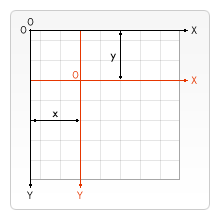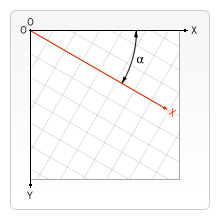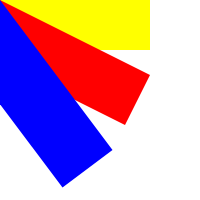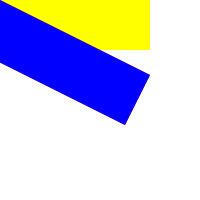# HTML5 Canvas ：变形和转换

## 位移

HTML5 Canvas 提供了 `translate` 方法，可重新映射画布上的 (0,0) 位置，再次绘制图形时，位置会相对新原点进行绘制，从而产生位移的效果。``````// 重新映射画布上的 (0,0) 位置。
// x:添加到水平坐标（x）上的值
// y:添加到垂直坐标（y）上的值
context.translate(x, y);
``````

``````var canvas=document.getElementById("myCanvas");
var ctx=canvas.getContext("2d");

ctx.fillRect(30,30,60,60);
ctx.translate(70,70);
ctx.fillRect(30,30,60,60);
``````## 缩放

HTML5 Canvas 提供了 `scale` 方法用来增减图形的单位绘图因子在 canvas 中包含的像素数目，对形状、位图进行缩小或者放大。

``````// 缩放当前绘图
//scalewidth:缩放当前绘图的宽度 (1=100%, 0.5=50%, 2=200% ...)
//scaleheight:缩放当前绘图的高度 (1=100%, 0.5=50%, 2=200% ...)
context.scale(scalewidth, scaleheight);
``````

``````var canvas=document.getElementById("myCanvas");
var ctx=canvas.getContext("2d");

ctx.fillRect(10,10,10,10);
ctx.scale(3, 3);
ctx.fillRect(10,10,10,10);
ctx.scale(1, 3);
ctx.fillRect(10,10,10,10);
``````## 旋转

HTML5 Canvas 提供了 `rotate ` 方法来旋转坐标轴，再次绘制图形时，位置会相对新坐标轴进行绘制，从而产生旋转的效果。``````// 旋转当前的绘图
// angle:旋转角度，以弧度计。
// 如需将角度转换为弧度，请使用 degrees*Math.PI/180 公式进行计算。
// 例如需旋转 5 度，可规定下面的公式：5*Math.PI/180。
context.rotate(angle);
``````

``````var canvas=document.getElementById("myCanvas");
var ctx=canvas.getContext("2d");

ctx.fillRect(20,10,100,30);
ctx.rotate(45 * Math.PI / 180); //旋转45度
ctx.fillRect(60,0,100,30);
``````## 变形

``````// 替换当前的变换矩阵
//                 a c e
// 变换矩阵的描述： [ b d f ]
//                 0 0 1
// a:水平缩放
// b:水平倾斜
// c:垂直倾斜
// d:垂直缩放
// e:水平移动
// f:垂直移动
ctx.transform(a, b, c, d, e, f);
``````

``````var canvas=document.getElementById("myCanvas");
var ctx=canvas.getContext("2d");

ctx.fillRect(50,30,50,50);
ctx.transform(1,1,0,1,0,0);
ctx.fillRect(50,50,50,50);
````````````var canvas=document.getElementById("myCanvas");
var ctx=canvas.getContext("2d");
ctx.fillStyle="yellow";
ctx.fillRect(0,0,150,50)

ctx.transform(1,0.5,-0.5,1,0,0);
ctx.fillStyle="red";
ctx.fillRect(0,0,150,50);

ctx.transform(1,0.5,-0.5,1,0,0);
ctx.fillStyle="blue";
ctx.fillRect(0,0,150,50);
``````## 设置变形矩阵

HTML5 Canvas 提供的 `setTransform` 方法用来重置并创建新的变换矩阵，它会覆盖到原有矩阵，并不会在之前变换的基础上进行变换。

``````// 设置新的变换矩阵
context.setTransform(a, b, c, d, e, f);
``````

``````var canvas=document.getElementById("myCanvas");
var ctx=canvas.getContext("2d");
ctx.fillStyle="yellow";
ctx.fillRect(0,0,150,50)

ctx.setTransform(1,0.5,-0.5,1,0,0);
ctx.fillStyle="red";
ctx.fillRect(0,0,150,50);

ctx.setTransform(1,0.5,-0.5,1,0,0);
ctx.fillStyle="blue";
ctx.fillRect(0,0,150,50);
``````